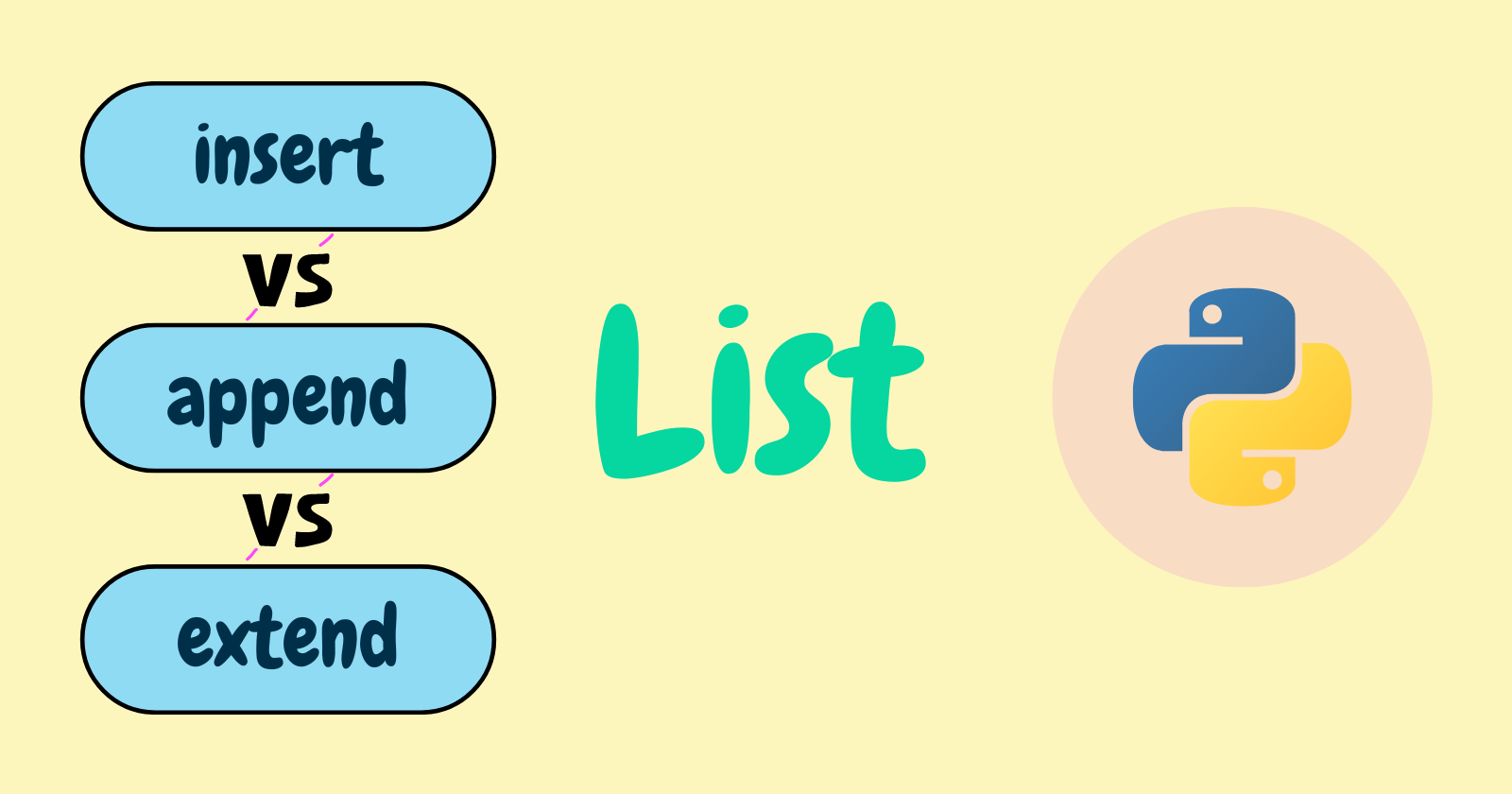## Difference Between insert(), append() And extend() In Python With Examples

List is one of Python’s built-in data structures for storing collections of modifiable and ordered data. Lists can hold a variety of data types, including strings, integers, and even lists.

Lists are mutable, which means they can be created and modified. Python provides some methods for modifying the data within the list.

This article will explain the distinctions between the list insert()append(), and extend() methods. We’ll see how they differ and how they’re used to modify the list.

## Methods Objective

Every Python developer would have worked with lists and used these methods periodically. List `insert()``append()`, and `extend()` are somewhat related because they are used to add elements to a list.

However, these methods are syntactically and programmatically distinct, each method has their own way of adding data to the list.

## List insert()

We already know what is the meaning of insert, it means putting or adding a particular element into the data. This method does the same work as its name meaning.

Python list `insert()` method helps to insert an element to the list at the desired position. This method takes two arguments, the first argument is the index at which the element is to be inserted and the second argument is the element that will be inserted.

`list.insert(index, element)`

Using the `insert()` method, we inserted the string `'Abhishek'` at the 1st index in `my_lst`.

## List append()

Python list `append()` method adds the element to the end of the list. It takes only one argument which is the element to be appended to the list.

`list.append(element)`

## List extend()

We’ve seen how we can use `append()` to add an element to the end of a list. List `extend()` does the same thing, but instead of adding a single element, it adds each item from the iterable, resulting in the list being extended.

`list.extend(iterable)` or `list.extend(['elem1', 'elem2', 'elem3'])`

We passed the list in the expression `friends.extend(['Abhishek', 'Yogesh'])` and as we can see the items became part of the original list.

## Difference

Time complexity refers to the computer time to run an algorithm.

## Conclusion

We’ve seen how the list insert()append(), and extend() methods differ from one another in this article. The goal of these methods is the same in that they add elements to the list, but they differ in how they add the elements to the list.

We’ve compared these methods using the code examples and here’s a recap of what we’ve learned

• `insert()` – This method is used to insert the element at the desired position in the list
• `append()` – This method is used to add the element to the end of the list.
• `extend()` – This method is used to add each item from the iterable to the end of the list.

🏆Other articles you might be interested in if you liked this one

That’s all for now

Keep Coding✌✌